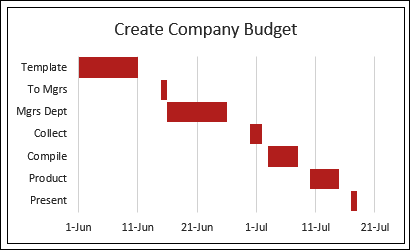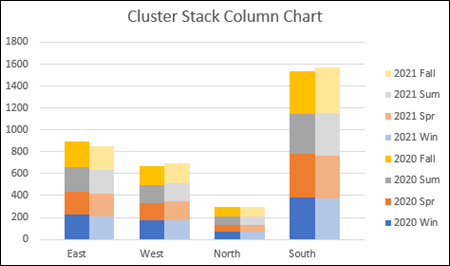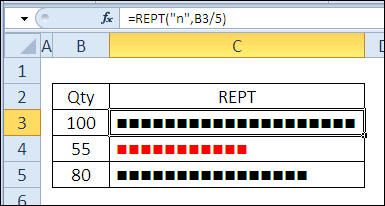# Excel Chart Videos & Tutorials 📈

Overview of Excel chart tutorials and tips, to help you get started, when creating new charts in Excel.

## Pie Charts

A pie chart shows amounts as a percentage of the total amount. Other charts, such as a bar chart or a column chart, are better for showing the differences between amounts.

If you need a pie chart, create a simple one, like the example below, without any special effects such as 3-D. Then, format the data labels, so the data is easier to understand.This short video shows the steps, and there are written steps on this page: Excel Pie Charts

## Gantt Charts

Although Excel doesn’t have a built in Gantt chart type, you can follow the steps below to create a simple project plan in Excel, with a Gantt chart timeline.

In the example shown below, I created a simple Gantt chart, to show the steps in working on a company budget. You can see when each step starts, and when it ends.## Combination Charts

If you use two different chart types in a single chart, it’s called a combination chart, like the line-column chart shown below.

In this example, each series is on a different axis, so you can compare the amounts (thousands), with the number of cases (hundreds). If both were on the same axis, the cases would be almost impossible to see, because those numbers as so small.## Cluster Stack Chart

Or, get really fancy, and make a cluster stack chart, which is a combination of a stacked column chart, and a clustered column chart. In the example shown below, the chart has:

• a Cluster of columns for each region (East, West, North, South)
• a Stack in each column, with a different coloured segment for each season in the year

You’ll find written steps and a video on this page: Excel Cluster Stack Chart## In-Cell Charts

You can even make charts in worksheet cells, by using the Excel REPT function. In the example shown below, I used the Wingdings font to create the squares, and the bar length is based on the number in column B. To create the column, for quantities less than 60, I added conditional formatting.## Video: Line Chart Target Range

In this video, see how to build an Excel line chart to show sales over six months, and show the target sales range in the chart's background. In the data, add the high and low values for the target range, and show that range in the background of the line chart.

To see how the chart works, you can download the sample file from the Excel Sample Files page. In the Charts and Graphics section, look for CH0008 – Show Target Range on Line Chart

## Chart Tutorials & Videos

Excel Chart Videos

Panel chart

Last updated: January 6, 2022 12:52 PM